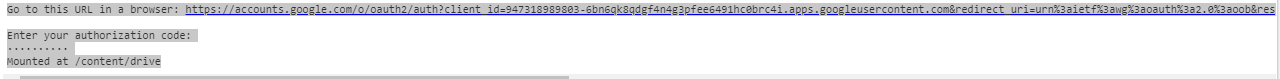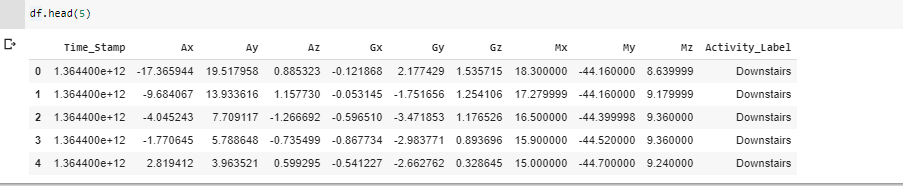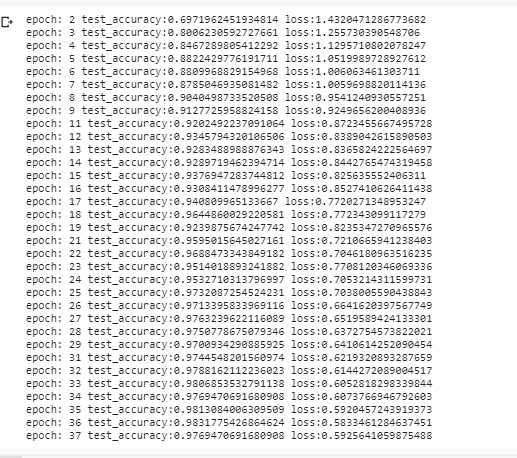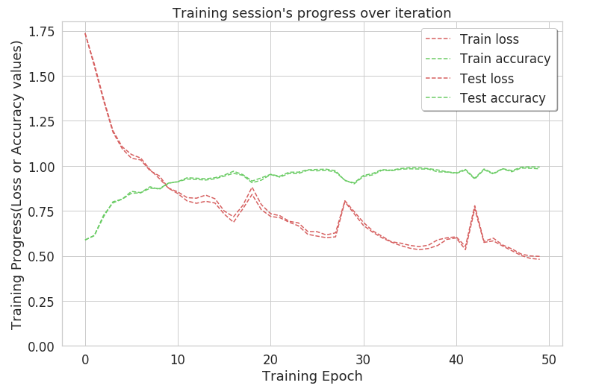GFG App
Open AppBrowser
Continue

# Human Activity Recognition – Using Deep Learning Model

Human activity recognition using smartphone sensors like accelerometer is one of the hectic topics of research. HAR is one of the time series classification problem. In this project various machine learning and deep learning models have been worked out to get the best final result. In the same sequence, we can use LSTM (long short term memory) model of the Recurrent Neural Network (RNN) to recognize various activities of humans like standing, climbing upstairs and downstairs etc.
LSTM model is a type of recurrent neural network capable of learning order dependence in sequence prediction problems. This model is used as this helps in remembering values over arbitrary intervals.
Activities:

• Walking
• Upstairs
• Downstairs
• Sitting
• Standing

Accelerometers detect magnitude and direction of the proper acceleration, as a vector quantity, and can be used to sense orientation (because direction of weight changes). GyroScope maintains orientation along a axis so that the orientation is unaffected by tilting or rotation of the mounting, according to the conservation of angular momentum.
Understanding the dataset:

• Both the sensors generate data in 3D space over time.
(‘XYZ’ represents 3-axial signals in X, Y, and Z directions.)
• The available data is pre-processed by applying noise filters and then sampled in fixed-width windows ie., each window has 128 readings.

Train and Test data were separated as
The readings from 80% of the volunteers were taken as training data and remaining 20% volunteers records were taken for test data. All the data is present in the folder downloaded using the link provided above.
Phases

• Choosing a dataset

• Dataset cleaning and data Preprocessing
• Choosing a model and building deep learned network model
• Exporting in Android Studio.

The IDE used for this project is Google Colaboratory which is the best of the times to deal with deep learning projects. Phase 1 was explained above as from where the dataset is downloaded. In this sequence to start with the project open a new notebook in Google Colaboratory first import all the necessary libraries.
Code: Importing Libraries

## Python3

 `import` `pandas as pd` `import` `numpy as np` `import` `pickle` `import` `matplotlib.pyplot as plt` `from` `scipy ``import` `stats` `import` `tensorflow as tf` `import` `seaborn as sns` `from` `sklearn ``import` `metrics` `from` `sklearn.model_selection ``import` `train_test_split`   `%``matplotlib inline `

Phase 2:
It is uploading dataset in the notebook, before doing that we need to mount the notebook on drive so that this notebook is saved on our drive and retrieved whenever required.

## Python3

 `sns.``set``(style``=``"whitegrid", palette``=``"muted", font_scale``=``1.5``)` `RANDOM_SEED ``=` `42`   `from` `google.colab ``import` `drive` `drive.mount(``'/content/drive'``)`

Output:

`You will see a pop up similar to one shown in the screenshot below, open the link and copy the authorization code and paste it in the authorization code bar and enter the drive will be mounted.`## Python3

 `from` `google.colab ``import` `files` `uploaded ``=` `files.upload()`

Now moving on to model building and training phase, we need to look for different models which can help in building better accuracy model. Here, LSTM model of Recurrent Neural Network is chosen. The image given below shows how the data looks.Phase 3:
It begins with the data pre-processing. It is the phase where ~90% of time is consumed in actual data science projects. Here, raw data is taken and converted in some useful and efficient formats.
Code: Data transformation is performed to normalize the data

## Python3

 `#transforming shape` `reshaped_segments ``=` `np.asarray(` `    ``segments, dtype ``=` `np.float32).reshape(` `    ``-``1` `, N_time_steps, N_features)`   `reshaped_segments.shape`

Code: Splitting the dataset

## Python3

 `X_train, X_test, Y_train, Y_test ``=` `train_test_split(` `    ``reshaped_segments, labels, test_size ``=` `0.2``, ` `    ``random_state ``=` `RANDOM_SEED)`

The test size is taken as 20% i.e. out of the total records 20% of records are used for test accuracy while rest is used for training model.
Number of classes = 6 (Walking, Sitting, Standing, Running, Upstairs and Downstairs)
Phase 4: In this phase model chosen is the LSTM model of RNN.
Code: Model building

## Python3

 `def` `create_LSTM_model(inputs):` `    ``W ``=` `{` `        ``'hidden'``: tf.Variable(tf.random_normal([N_features, N_hidden_units])),` `        ``'output'``: tf.Variable(tf.random_normal([N_hidden_units, N_classes]))` `    ``}` `    ``biases ``=` `{` `        ``'hidden'``: tf.Variable(tf.random_normal([N_hidden_units], mean ``=` `0.1``)),` `        ``'output'``: tf.Variable(tf.random_normal([N_classes]))` `    ``}` `    ``X ``=` `tf.transpose(inputs, [``1``, ``0``, ``2``])` `    ``X ``=` `tf.reshape(X, [``-``1``, N_features])` `    ``hidden ``=` `tf.nn.relu(tf.matmul(X, W[``'hidden'``]) ``+` `biases[``'hidden'``])` `    ``hidden ``=` `tf.split(hidden, N_time_steps, ``0``)`   `    ``lstm_layers ``=` `[tf.contrib.rnn.BasicLSTMCell(` `        ``N_hidden_units, forget_bias ``=` `1.0``) ``for` `_ ``in` `range``(``2``)]` `    ``lstm_layers ``=` `tf.contrib.rnn.MultiRNNCell(lstm_layers)`   `    ``outputs, _ ``=` `tf.contrib.rnn.static_rnn(lstm_layers, ` `                                           ``hidden, dtype ``=` `tf.float32)`   `    ``lstm_last_output ``=` `outputs[``-``1``]` `    ``return` `tf.matmul(lstm_last_output, W[``'output'``]) ``+` `biases[``'output'``]`

Code: Performing optimization using AdamOptimizer to modifier loss values wrt the variables to improve accuracy and reduce loss.

## Python3

 `L2_LOSS ``=` `0.0015` `l2 ``=` `L2_LOSS ``*` `\` `  ``sum``(tf.nn.l2_loss(tf_var) ``for` `tf_var ``in` `tf.trainable_variables())` `loss ``=` `tf.reduce_mean(tf.nn.softmax_cross_entropy_with_logits(` `    ``logits ``=` `pred_y, labels ``=` `Y)) ``+` `l2`   `Learning_rate ``=` `0.0025` `optimizer ``=` `tf.train.AdamOptimizer(learning_rate ``=` `Learning_rate).minimize(loss)` `correct_pred ``=` `tf.equal(tf.argmax(pred_softmax , ``1``), tf.argmax(Y,``1``))` `accuracy ``=` `tf.reduce_mean(tf.cast(correct_pred, dtype ``=` `tf.float32))`

Code: Performing 50 iterations of model training to get the highest accuracy and reduced loss

## Python3

 `# epochs is number of iterations performed in model training.` `N_epochs ``=` `50`  `batch_size ``=` `1024`   `saver ``=` `tf.train.Saver()` `history ``=` `dict``(train_loss``=``[], train_acc``=``[], test_loss``=``[], test_acc``=``[])` `sess ``=` `tf.InteractiveSession()` `sess.run(tf.global_variables_initializer())` `train_count ``=` `len``(X_train)`   `for` `i ``in` `range``(``1``, N_epochs ``+` `1``):` `    ``for` `start, end ``in` `zip``(``range``(``0``, train_count, batch_size), ` `                          ``range``(batch_size, train_count ``+` `1``, batch_size)):` `        ``sess.run(optimizer, feed_dict``=``{X: X_train[start:end],` `                                       ``Y: Y_train[start:end]})` `    ``_, acc_train, loss_train ``=` `sess.run([pred_softmax, accuracy, loss], feed_dict``=``{` `        ``X: X_train, Y: Y_train})` `    ``_, acc_test, loss_test ``=` `sess.run([pred_softmax, accuracy, loss], feed_dict``=``{` `        ``X: X_test, Y: Y_test})` `    ``history[``'train_loss'``].append(loss_train)` `    ``history[``'train_acc'``].append(acc_train)` `    ``history[``'test_loss'``].append(loss_test)` `    ``history[``'test_acc'``].append(acc_test)`   `    ``if` `(i !``=` `1` `and` `i ``%` `10` `!``=` `0``):` `        ``print``(f``'epoch: {i} test_accuracy:{acc_test} loss:{loss_test}'``)` `predictions, acc_final, loss_final ``=` `sess.run([pred_softmax, accuracy, loss], ` `                                              ``feed_dict``=``{X: X_test, Y: Y_test})` `print``()` `print``(f``'final results : accuracy : {acc_final} loss : {loss_final}'``)` `   `

Output:So, with this approach the accuracy reaches nearly ~1 at 50th iteration. This indicates that most of the labels are identified clearly by this approach. To get the exact count of correctly identified activities confusion matrix is created.
Code: Accuracy graph

## Python3

 `plt.figure(figsize``=``(``12``,``8``))`   `plt.plot(np.array(history[``'train_loss'``]), "r``-``-``", label``=``"Train loss")` `plt.plot(np.array(history[``'train_acc'``]), "g``-``-``", label``=``"Train accuracy")`   `plt.plot(np.array(history[``'test_loss'``]), "r``-``-``", label``=``"Test loss")` `plt.plot(np.array(history[``'test_acc'``]), "g``-``-``", label``=``"Test accuracy")`   `plt.title("Training session's progress over iteration")` `plt.legend(loc ``=` `'upper right'``, shadow ``=` `True``)` `plt.ylabel(``'Training Progress(Loss or Accuracy values)'``)` `plt.xlabel(``'Training Epoch'``)` `plt.ylim(``0``)`   `plt.show()`Confusion Matrix: A confusion matrix is no less then a 2D matrix unlike it helps calculating exact count of activities correctly identified. In other words, it describes the performance of the classification model on the set of test dataset.
Code: Confusion matrix

## Python3

 `max_test ``=` `np.argmax(Y_test, axis``=``1``)` `max_predictions ``=` `np.argmax(predictions, axis ``=` `1``)` `confusion_matrix ``=` `metrics.confusion_matrix(max_test, max_predictions)`   `plt.figure(figsize``=``(``16``,``14``))` `sns.heatmap(confusion_matrix, xticklabels ``=` `LABELS, yticklabels ``=` `LABELS, annot ``=``True``, fmt ``=` `"d")` `plt.title("Confusion Matrix")` `plt.xlabel(``'Predicted_label'``)` `plt.ylabel(``'True Label'``)` `plt.show()`

This is the complete description about the project so far. It can be built using other models like CNN or machine learning models like KNN.

My Personal Notes arrow_drop_up